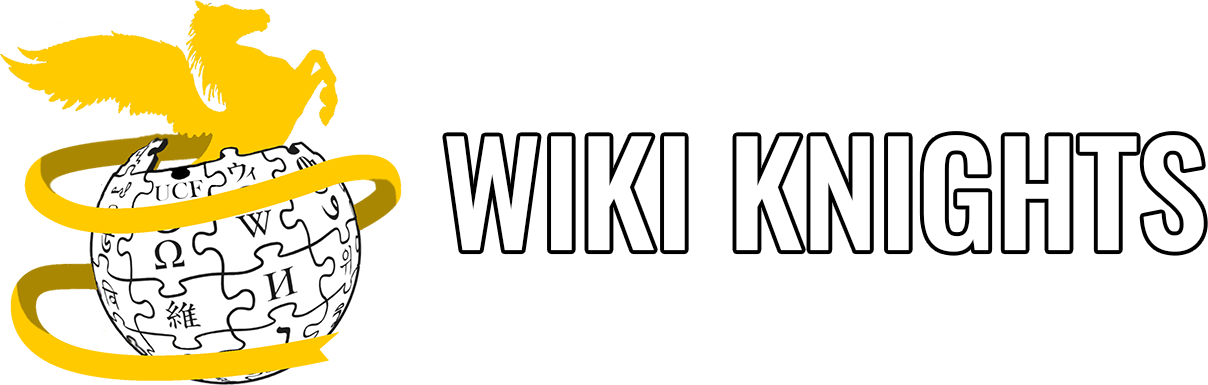# Conditional Statement Practice

Created by Jerrett Longworth in February 2022.

How can you check if something is true or not, and can you get your code to act differently depending on those conditions? Yes, you can! Let’s get some practice using conditional statements.

1. Whenever Bob is in class, the variable `class` is set to 1, and 0 otherwise. If Bob is doing homework, the variable `homework` is set to 1, and 0 otherwise. Create an expression using boolean operators and the provided variables that would evaluate to true if Bob is doing their homework in class.

2. Given the following program, what will be the output?

``````#include <stdio.h>

int main(void)
{
int x = 5;
int y = 17;

if (x > y)
{
printf("This is statement #1!\n");
}
else
{
printf("This is statement #2!\n");
}

return 0;
}``````
1. Given the following program, what will be the output?
``````#include <stdio.h>

int main(void)
{
int x = 13;
int y = 6;
int z = 15;

if (x < z)
{
printf("This is statement #1!\n");
}
else if (x > y)
{
printf("This is statement #2!\n");
}
else
{
printf("This is statement #3!\n");
}

return 0;
}``````
1. From the program in the previous problem, how would the output change if the “`else if`” changed to “`if`” (or would it change at all)?

2. Given the following program, what will be the output?

``````#include <stdio.h>

int main(void)
{
int x = 5;
int y = 17;

if (!(x > y))
{
printf("This is statement #1!\n");
}
else
{
printf("This is statement #2!\n");
}

return 0;
}``````
1. Given the following program, what will be the output?
``````#include <stdio.h>

int main(void)
{
int x = 19;
int y = 100;
int z = 2;

if (y > z || y < x)
{
printf("This is statement #1!\n");
}
else
{
printf("This is statement #2!\n");
}

return 0;
}``````
1. Given the following program, what will be the output?
``````#include <stdio.h>

int main(void)
{
int x = 213;
int y = 912;
int z = 1021;

if (x == y || z < x)
{
printf("This is statement #1!\n");
}
else if (y <= z && (x - 213 == 1 || y != 213))
{
printf("This is statement #2!\n");
}
else
{
printf("This is statement #3!\n");
}

return 0;
}``````

## Challenge problems

In some curricula, conditions are covered before functions, so these following questions are considered “challenge problems.” If you have already studied functions, these additional problems are excellent exercises to get more familiar with both conditionals and functions.

1. Given the following program, what will be the output?
``````#include <stdio.h>

int fun_function(int y)
{
if (y > 10)
{
return 5123;
}
else
{
return 64;
}
}

int main(void)
{
int x = 8511;
int y = 754;
int z = 27;

if (x > z && fun_function(z) >= 65)
{
printf("This is statement #1!\n");
}
else
{
printf("This is statement #2!\n");
}

return 0;
}``````
1. Given the following program, what will be the output?
``````#include <stdio.h>

int funny_function(int x)
{
x = x / 2;

if (x > 50)
{
printf("This is statement #1!\n");
}
else
{
printf("This is statement #2!\n");
}

return x;
}

int main(void)
{
int x = 4;
int y = 111;
int z = 1520;

if (z > y || funny_function(z) > 65)
{
printf("This is statement #3!\n");
}
else
{
printf("This is statement #4!\n");
}

return 0;
}``````
1. Given the following program, what will be the output?
``````#include <stdio.h>

int funny_function(int z)
{
z = z / 2;

if (z > 50)
{
printf("This is statement #1!\n");
}
else
{
printf("This is statement #2!\n");
}

return z;
}

int main(void)
{
int x = 6854;
int y = 111;
int z = 1520;

if (z > y && funny_function(z) < 65)
{
printf("This is statement #3!\n");
}
else
{
printf("This is statement #4!\n");
}

return 0;
}``````